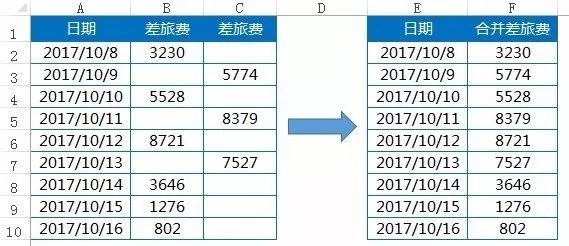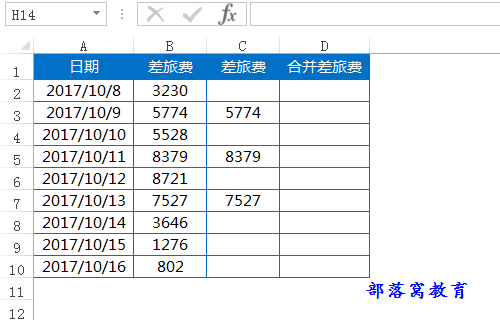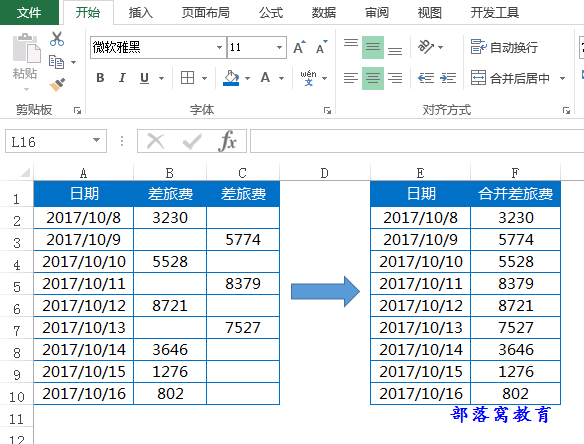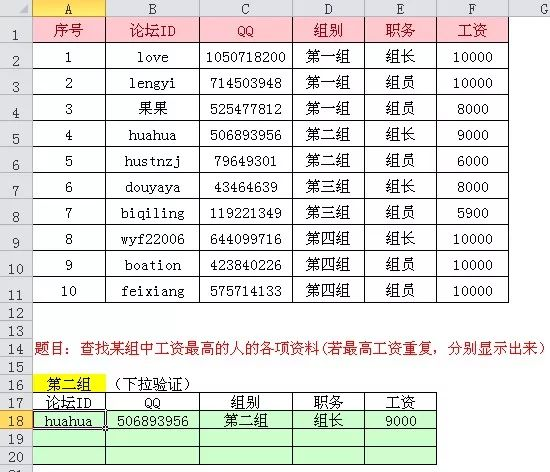# Excel教程：万能筛选公式，提高你的工作效率20%！01excel跳过空单元格操作技巧如下：02

INDEX+SMALL函数构造筛选公式A18输入公式，按下ctrl+shift+enter组合键完成数组公式的输入，然后右拉下拉复制公式。
=INDEX(\$B:\$F,SMALL(IF(\$F\$2:\$F\$11=MAX((\$D\$2:\$D\$11=\$A\$16)*\$F\$2:\$F\$11),ROW(\$2:\$11),4^8),ROW(A1)),COLUMN(A1))&""

INDEX(\$B:\$F,行部分,COLUMN(A1))&""
INDEX+SMALL函数构造出来的筛选公式，经典在于获取出相应的行。剖析公式一般从内到外，用F9键逐一查看运算结果。

SMALL部分，获取行号，剖析如下：

1.MAX部分：

MAX((D2:D11=A16)*F2:F11))

D2:D11=A16，判断D列的组别和A16组别是否相等，得到FALSE和TRUE构成的逻辑数组。

(D2:D11=A16)*F2:F11，计算结果将符合条件的true对应的数字取出来：

{0;0;0;9000;6000;0;0;0;0;0}

2.IF部分：

IF(条件,是,否)

IF(F2:F11=9000,ROW(\$2:\$11),4^8)

3.SMALL部分：

SMALL(最大行号和符合条件的行号，ROW(A1))

SMALL({65536;65536;65536;5;65536;65536;65536;65536;65536;65536},ROW(A1))，结果是5。

INDEX部分解析

INDEX(区域,行,列)

INDEX(\$B:\$F,5,COLUMN(A1))，返回B:F列这个区域的第五行第一列，对应的单元格就是B5单元格。

&""是什么意思呢? &是个文本粘贴符，后面的""是表示空白文本，就等于在后面强制性的把(0)粘贴成了空白文本。